Language :

Quantitative Aptitude Expected Questions for SBI Clerk Mains 2019Question 1 A and B working separately can do a piece of work in 10 days and 15 days respectively. It they work on alternate days beginning with A, in how many days will the work be completed?   A and B working separately can do a piece of work in 10 days and 15 days respectively. It they work on alternate days beginning with A, in how many days will the work be completed?  Read Less
 A 18 daysB 13 daysC 12 daysD 6 daysE 14 days
 Question 2 A can do as much work as B and C together can do. A and B can together do a piece of working in 9 hours 36 minutes and C can do it in 48 hours. The time (in hours) that B needs to do the work alone, is   A can do as much work as B and C together can do. A and B can together do a piece of working in 9 hours 36 minutes and C can do it in 48 hours. The time (in hours) that B needs to do the work alone, is  Read Less
 A 18B 24C 30D 12E 16
 Question 3 Two guns are fired from the same place at an interval of 6 minutes. A person approaching the place observes that 5 minutes 52 seconds have elapsed between the hearing of the sound of the two guns. If the velocity of the sound is 330 m/s, the man was approaching that place at what speed (in kmph)?   Two guns are fired from the same place at an interval of 6 minutes. A person approaching the place observes that 5 minutes 52 seconds have elapsed between the hearing of the sound of the two guns. If the velocity of the sound is 330 m/s, the man was approaching that place at what speed (in kmph)?  Read Less
 A 24B 27C 30D 36E 46
 Question 4 The ratio of area of an equilateral triangle and a rectangle is 6 : 13. The side of equilateral triangle is 6/7of diameter of a circle whose area is 616 m². Find the area of rectangle (in m²). (Take π = 22/7)  The ratio of area of an equilateral triangle and a rectangle is 6 : 13. The side of equilateral triangle is 6/7of diameter of a circle whose area is 616 m². Find the area of rectangle (in m²). (Take π = 22/7) Read Less
 A 316 √3B 312√3C 321√3D 324√3E 212√3
 Question 5 Two bags A and B contain 7 red and 6 blue balls respectively. Some blue balls from bag B are taken out and kept into bag A. If probability of selecting two blue ball from bag A is 1/15, find the number of blue balls drawn from bag B.   Two bags A and B contain 7 red and 6 blue balls respectively. Some blue balls from bag B are taken out and kept into bag A. If probability of selecting two blue ball from bag A is 1/15, find the number of blue balls drawn from bag B.  Read Less
 A 2B 4C 3D 5E 6
 Question 6 Directions (06-10): In each of the following questions two equations are given. You have to solve the equations and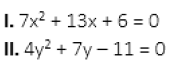Directions (06-10): In each of the following questions two equations are given. You have to solve the equations andRead Less
 A if x < yB if x ≤ yC  relationship between x and y cannot be determinedD if x ≥ yE ifx>y
 Question 7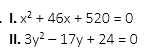Read Less
 Aif x < yBif x ≤ yC relationship between x and y cannot be determinedDif x ≥ yEifx>y
 Question 8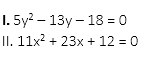Read Less
 Aif x < yBif x ≤ yC relationship between x and y cannot be determinedDif x ≥ yEifx>y
 Question 9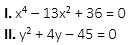Read Less
 A if x < yB if x ≤ yC  relationship between x and y cannot be determinedD if x ≥ yE ifx>y
 Question 10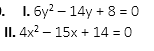Read Less
 Aif x < yBif x ≤ yCrelationship between x and y cannot be determinedDif x ≥ yEifx>y

## SBI Clerk - ONLINE TEST

IBT Institute offer you the most trusted and highly recommended Test Series for SBI Clerk Exam. 8 out of every 10 candidates selected in SBI Clerk last year opted for IBT Online Test Series.

## SBI Clerk - CLASS ROOM COURSE

Keeping in mind the SBI Clerk Exam, IBT has launched Special batches for SBI Clerk Exam to guide you through your preparations. Seats filling fast. Don’t miss out!!Correspondence Course

For SBI PO Exam (Pre+Main)

9 Books , 350 Tests

52 Assignments , Online Doubts